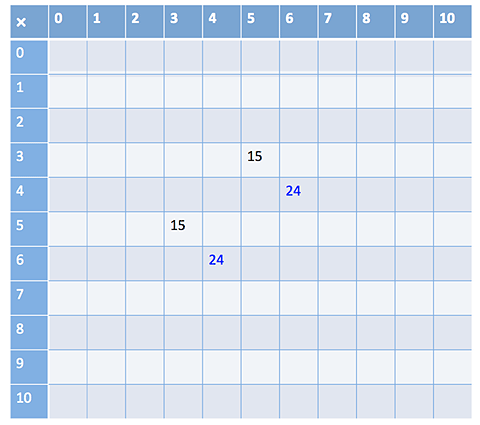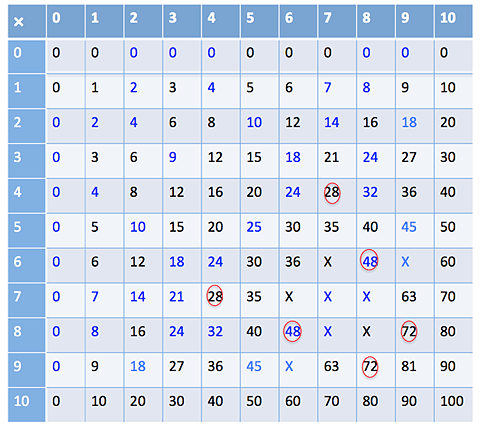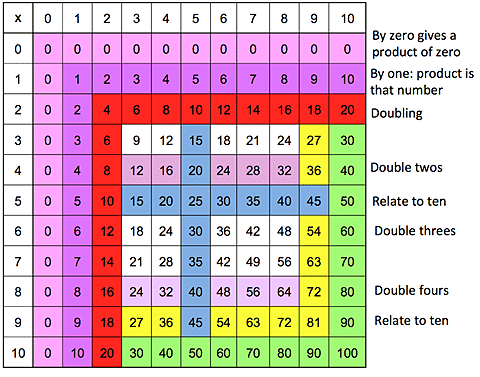Home > Mental computation > Activities > What do I know?

# What do I know?

Developing an understanding of the properties of multiplication (such as the properties of 0, 1, commutativity) reduce the number of facts students need to remember.

### What do I know?

Materials: Blank multiplication grid and two 10-sided (0-9) dice per pair, coloured pencils.

• In pairs, students take turns to roll the dice and generate a multiplication fact.
For example, player A rolls (5, 3) and player B says "5 threes are 15, and 3 fives are 15".
• Player B records 15 in the appropriate cells on the grid, using a coloured pencil.Sample multiplication fact grid.

• If a player takes a long time to work out the product, it is recorded in the cell with a circle around it. If a player does not know then a cross is put in the cell.Completed multiplication fact grid.

• Play continues until all the cells are filled. Students should record the facts for 10 as they cannot be generated using the dice.
• Students discuss and list some of the strategies used for the facts that were tricky to work out.

You can watch the video What Do I Know?

List some of the strategies students used for the facts that were tricky to work out (e.g. using 4 $$\times$$ 4 and doubles to work out 8 $$\times$$ 4).

List the facts that were not known. Discuss and list some strategies that might help learn these facts (e.g. 7 $$\times$$ 4 = 5 $$\times$$ 4 + 2 $$\times$$ 4).

Which facts are known without having to think? Shade these on a blank multiplication grid.

Which facts can be worked out using doubling or thinking of tens facts? Shade these on the grid.